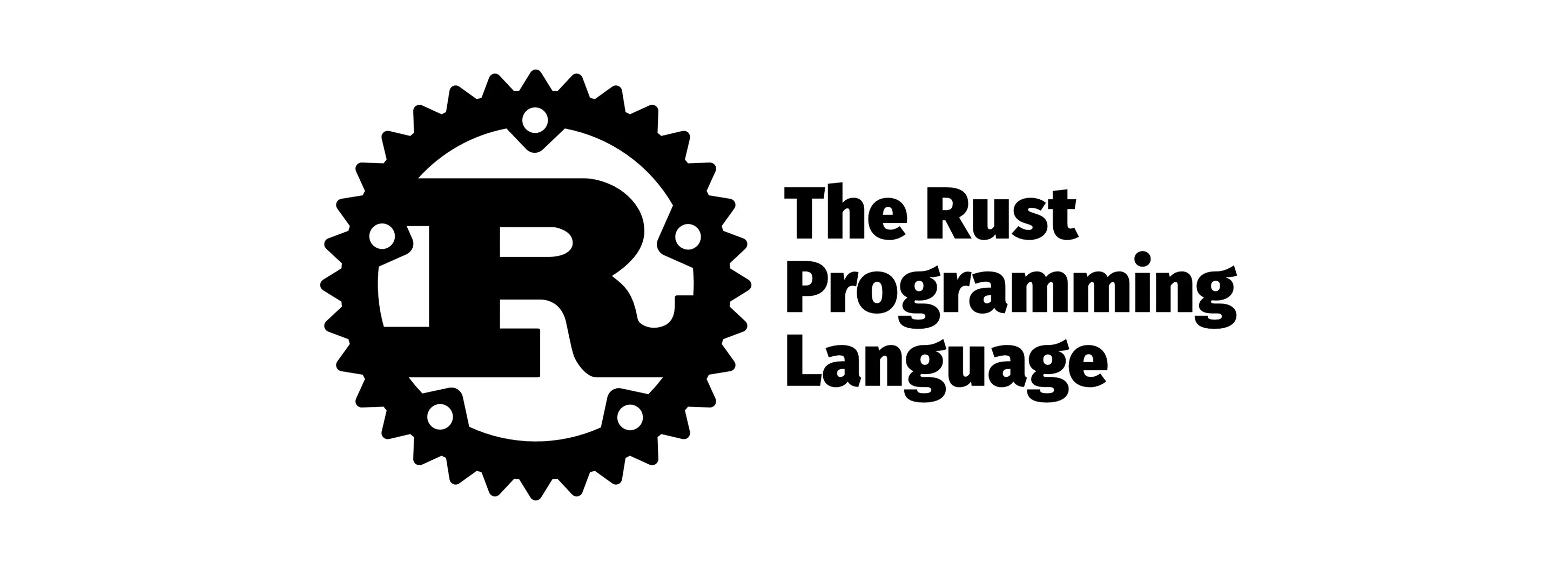Back## 变量声明

Rust 使用 let 关键字声明变量，和其他新兴语言一样，默认情况下是 Immutable 的。需要使用 mut 关键字使其可变。

fn main() {
let x = 5;
println!("The value of x: {}", x);
let mut y = 5;
println!("The value of y: {}", y);
}

const MAX_POINTS: u32 = 100_000; // compile-time constant

fn main() {
const STRING: &str = "123123";
println!("The value of MAX_POINTS: {}", MAX_POINTS);
}

let a = 1;
let a = 100;

## 注释

// 单行注释

/*
* 多行注释
* /*
*  * 可以嵌套
*  */
*/

///
/// 文档注释
///

## 基本类型

### 整数类型

8 位i8u8
16 位i16u16
32 位i32u32
64 位i64u64
128 位i128u128

Rust 的基本类型关键字非常简洁易懂，直接就是类型缩写 + 所占空间，不必纠结 int long long long long int short 的意义。

isizeusize 即根据平台使用不同的长度，如 32 位架构上，就占 32 位 ，最常用来存数组下标。

Rust 也支持不同进制的整数字面量：

### 浮点类型

Rust 的浮点数遵循 IEEE 754 标准。具有 f32f64 两种长度可选。默认为 f64

### 字符

let a = 'a'
let alpha = 'α'
let emoji = '😄' // 大多数 emoji 都可以存为字符

### 复合类型

• 数组：[1, 2, 3]

• Rust 的数组索引和多数 C-like 语言一样，从 0 开始
• 数组的长度是固定的，所以类型注解应写成 let arr: [i32; 5] = [1,2,3,4,5]
• 多个同样的值可以使用 [0; 5] 这种语法，相当于 [0, 0, 0, 0, 0,]
• 在编译时，Rust 能够检测部分数组越界。如 let a = [1,2,3]; a; 会直接不过编译。
• 在运行时，若数组越界了，将会直接 panic 退出程序，而不是继续执行。
• 元组：(1, true)

• 类型注解类似这样：(i32, bool)

• 当元素多于 3 个时，建议使用结构体定义。

• 可以直接访问对应的类型： x.0 x.1

• 解构声明：let (x, y, z) = (500, 6.4, 1);

### 字面量后缀

let a = 100i64;
let b = 100i32;
let c = 100i8;

let d = 10.123f32;
let f = 10.112312312f64;

## 函数

fn function() { // 隐式返回 Unit 类型 ()
println!("Hello Function!");
another_function();
}

fn another_function() {
println!("Another function.");
}

fn main() {
another_function(5, 'g');
}

fn another_function(x: i32, lable: char) {
println!("The value of x is: {}{}", x, lable);
}

Rust 刻意不支持默认参数，也不支持函数重载。

Rust 允许将不写分号的语句直接返回，而不用写 return

fn return_no_semicolon() -> i32 {
123123
}

fn return_no_semicolon() -> i32 = 123123

## 控制流

### 条件

#### if-else

[dependencies]
rand = "0.8.0"
use rand::{thread_rng, Rng}; // 导包

fn main() {
// 这里的 ..= 是闭区间, 也就是说包括 1 和 100
// 与之对应的有 .. 是左闭右
if num < cmp_num {
println!("{num} is greater than {cmp_num}!")
} else {
println!("{num} is not greater than {cmp_num}!")
}
}

Rust 的条件必须是布尔类型，以下代码会报错：

if 1 {}

#### if-else-if

// 后面将会省略 main 函数和生成 num 的代码
if num % 3 == 0 {
println!("{num} is is divisible by 3!")
} else if num % 4 == 0 {
println!("{num} is is divisible by 4!")
} else if num % 5 == 0 {
println!("{num} is is divisible by 5!")
} else {
println!("{num} is not divisible by 3, 4, 5.")
}

#### match

match num {
1 => println!("1"),
2 => println!("2"),
_ => println!("Other number"),
}

match 要求分支必须是穷尽的，常常用来处理枚举。后面讲到枚举的时候再详细介绍。

#### 作为表达式

fn main() {
let condition = true;
let number = if condition { 5 } else { 6 };

println!("The value of number is: {}", number);
}

### 循环

#### while

let mut count = 0;

while count < 5 {
count += 1;
println!("Again! {count}");
}

#### break & continue

break 用于跳出整个循环。continue 用于跳过本次循环。

fn main() {
let mut count = 0;

while count < 5 {
count += 1;
print!("{count} ");
if count == 1 {
continue;
}
if count == 3 {
break;
}
}
}

#### label

fn main() {
let mut count = 0;
'counting_up: loop {
println!("count = {}", count);
let mut remaining = 10;

loop {
println!("remaining = {}", remaining);
if remaining == 9 {
break;
}
if count == 2 {
break 'counting_up;
}
remaining -= 1;
}

count += 1;
}
println!("End count = {}", count);
}

/*
Output:
count = 0
remaining = 10
remaining = 9
count = 1
remaining = 10
remaining = 9
count = 2
remaining = 10
End count = 2
*/

#### for

for count in 1..=5 {
println!("Again! {count}");
}

// rev() 用于逆序, 此处会从 5 倒数到 1
for count in (1..=5).rev() {
println!("Again! {count}");
}

#### foreach

let a = [10, 20, 30, 40, 50];
for element in a.iter() {
println!("The value is {element}");
}